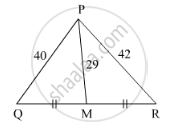Share

# Seg Pm is a Median of ∆Pqr. If Pq = 40, Pr = 42 and Pm = 29, Find Qr. - Geometry

ConceptApollonius Theorem

#### Question

Seg PM is a median of ∆PQR. If PQ = 40, PR = 42 and PM = 29, find QR.

#### SolutionIn ∆PQR, point M is the midpoint of side QR.

$QM = MR = \frac{1}{2}QR$

${PQ}^2 + {PR}^2 = 2 {PM}^2 + 2 {QM}^2 \left( \text{by Apollonius theorem} \right)$
$\Rightarrow \left( 40 \right)^2 + \left( 42 \right)^2 = 2 \left( 29 \right)^2 + 2 {QM}^2$
$\Rightarrow 1600 + 1764 = 1682 + 2 {QM}^2$
$\Rightarrow 3364 - 1682 = 2 {QM}^2$
$\Rightarrow 1682 = 2 {QM}^2$
$\Rightarrow {QM}^2 = 841$
$\Rightarrow QM = 29$
$\Rightarrow QR = 2 \times 29$
$\Rightarrow QR = 58$

Hence, QR = 58.

Is there an error in this question or solution?

#### APPEARS IN

Balbharati Solution for Balbharati Class 10 Mathematics 2 Geometry (2018 to Current)
Chapter 2: Pythagoras Theorem
Problem Set 2 | Q: 17 | Page no. 44
Solution Seg Pm is a Median of ∆Pqr. If Pq = 40, Pr = 42 and Pm = 29, Find Qr. Concept: Apollonius Theorem.
S